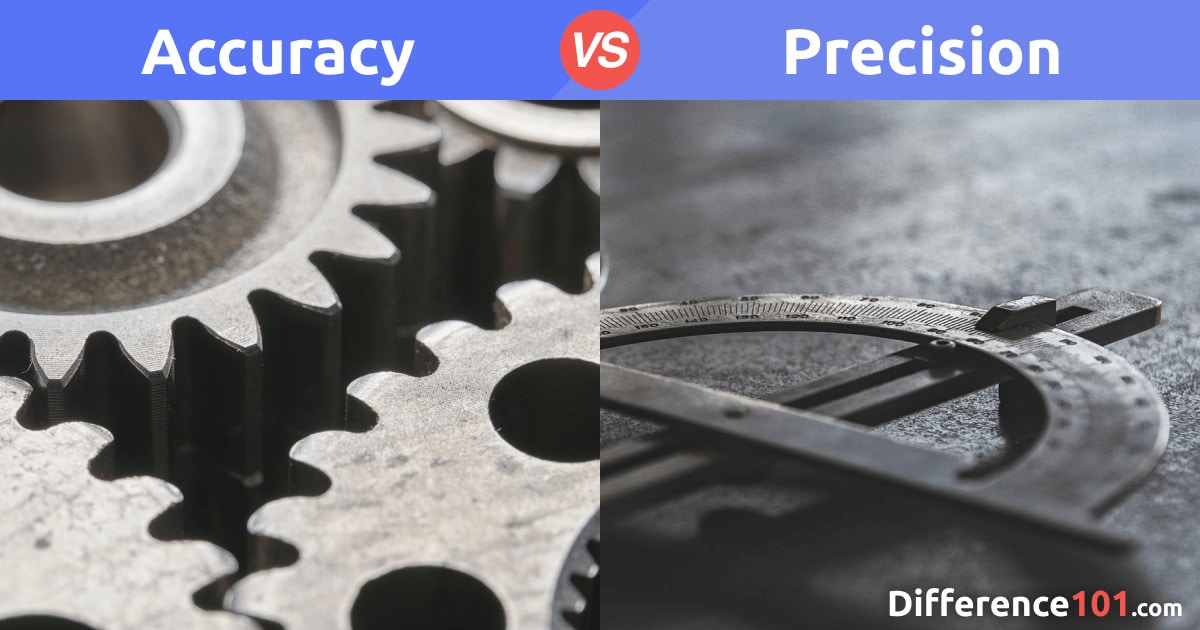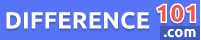# Accuracy vs. Precision: What’s The Difference Between Accuracy and Precision?

The main difference between Accuracy and Precision is that accuracy is the closeness of a particular measurement to the standard value, whereas precision is the closeness of a set of presented values. Simply put, accuracy measures correctness while precision measures exactness or the extent of reproducibility. To the layman, accuracy and precision could be used interchangeably but to a person that requires scientific measurements, they are two different things. To describe how precise or accurate a value is, scientists use a simple calculation known as the percent error.The main difference between Accuracy and Precision is that accuracy is the closeness of a particular measurement to the standard value, whereas precision is the closeness of a set of presented values.

Simply put, accuracy measures correctness while precision measures exactness or the extent of reproducibility.

To the layman, accuracy and precision could be used interchangeably but to a person that requires scientific measurements, they are two different things.

To describe how precise or accurate a value is, scientists use a simple calculation known as the percent error.

## What Is Accuracy?

Accuracy is a term used to denote the extent to which a value complies with the standard measurement. It measures the correctness of a result when compared to the absolute value.

This means that the closer a measurement is to the standard value, the higher the degree of accuracy.

Accuracy depends on the manner in which data is collected.

????  Protic vs. Aprotic: What's the Difference Between Protic and Aprotic?

## What Is Precision?

When measurements are repeated, precision shows the consistency in the results obtained.

Precision can then be said to be the degree of uniformity or repeatability in measurements. It is a measure of excellence when the results of a technique or operation are obtained.

When precision is high, it means the variation between the measurements was limited, and when values are precise they differ from one another due to random error, which is a type of observational error.

## What Are The Similarities Between Accuracy and Precision?

When taking measurements, whether statistical or scientific, for engineering or research purposes, accuracy and precision are very important.

Both show the closeness of a measurement to an actual value. Accuracy shows the closeness of a measurement to a known standard, and precision shows the reproducibility of a set of measurements and does not matter how far they are from the standard.

## Accuracy vs. Precision in Chemistry

In the process of taking scientific measurements, accuracy and precision are very important.

Accuracy measures the closeness of a measurement to its true value and is imperative as poor data processing, bad equipment, and human error could generate results that are far from the truth, and therefore not accurate.

Precision, however, measures the closeness of a series of values of the same factor in relation to each other.

Therefore, when measurements are not precise, random errors may not be identified and a wide result might be obtained.

## Accuracy and Precision Worksheet

To determine whether a measurement is adequately accurate or precise, the percentage error calculation can be used. It is very important for a measurement to be both accurate and precise.

## Accuracy And Precision Key Differences:

The main differences between accuracy and precision are:

### Definition

Accuracy is the extent of agreement between the actual measurement and the standard measurement, whereas precision is the extent of variability between the values of several measurements of a particular factor.

????  Starch vs. Glycogen vs. Cellulose: What is the difference between Starch and Glycogen and Cellulose?

### What They Stand For?

Accuracy is the closeness of agreement of a result to the standard measurement, while precision measures the closeness of these values to each other.

### Degree

While accuracy measures the degree of conformity of a measurement to the standard value, precision measures the degree of reproducibility and consistency of the values.

### Factors

Accuracy depends on one factor, where precision is based on multiple factors.

### What They Focus On?

Accuracy is concerned with errors due to faulty instruments called systematic errors, while precision is focused on random errors.

### What They Measure?

Accuracy measures statistical bias whereas precision measures the statistical variation.

## Accuracy and Precision Examples:

### Example #1 of Accuracy and Precision

When you repeatedly throw an arrow with the aim of hitting the center of the bull’s eye, Accuracy is how close your arrow gets to your target: the bull’s eye center.

In this case, Precision is when you continue to hit the same spot over and over again and it may not necessarily be the center of the bull’s eye.

### Example #2 of Accuracy and Precision

Here is another example:

If the correct answer to a problem is 2 and five students got

2, 2.2, 2.5, 1.9 and 1.95,

you can say that there is Accuracy in their answer because the values are all close to 2, which is the correct answer.

However, if the correct answer is 6 and one person gets 5.9 and the others got much higher or lower values, then the student who got 5.9 is more accurate.

On the other hand, if the five students each got

10, 10.1, 9.8, 11, and 9,

the values are Precise or close to each other but not accurate as they cannot be compared to 2, which is the correct answer.

When measurements are both accurate and precise, it means they are both reproducible and also very close to the standard values.

????  Qualitative vs. Quantitative: Difference, Similarity and Examples

## Conclusion

In conclusion, it should be noted that if a measurement has high accuracy and precision, the results obtained will be error-free. However, if the actual measurement is not accurate but it is precise, the result will not be close to the standard.

Also, if a measurement is accurate but not precise, there will be great variations in the measurements. If there is neither precision nor accuracy in the actual measurement, then the results obtained will neither be correct nor exact.

When taking any measurement, accuracy and precision should be your goal as they are very pertinent to good measurements in science.

Image Courtesy:

• Photo by Bill Oxford on Unsplash
• Photo by Andrea Sonda on Unsplash
We will be happy to hear your thoughtsLime vs. Lemon: What’s the difference?